telescopeѲptics.net          ▪▪▪▪                                             CONTENTS

10.2.4.7. Houghton-Cassegrain telescope: designing

In designing a two-mirror full aperture Houghton catadioptric telescope, the most important additional factor with respect to a single-mirror system is that of the secondary mirror. It induces both spherical aberration and coma of the opposite sign to that of the primary. In effect, it changes the amount of aberration to correct. The new corrector requirement in this respect is obtainable by correcting the system aberration coefficient for spherical aberration and coma for the effect of the secondary.

For the conventional, symmetrical Houghton corrector, the secondary-to-primary mirror aberration contribution for the lower-order spherical is given by: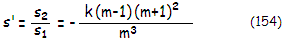with s1 and s2 being the aberration coefficients of spherical aberration for the primary and secondary, respectively and, as before, k is the relative height of the marginal ray at the secondary in units of the primary's semi-diameter and m is the secondary magnification. Since k=(1+η)/(m+1), the relation s'=(1+η)(m2-1)/m3 can be more practical for systems with the fixed value of back focal length in units of primary's f.l., η.

For the lower-order coma, the secondary contribution relative to that of the primary mirror is: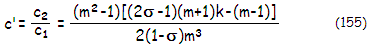with c1 and c2 being the coma aberration coefficients for the primary and secondary, respectively, and σ the stop (corrector) to primary separation in units of the primary radius of curvature (this means that 2σ-1 is numerically negative for σ<0.5, which is required for compact systems).

Both, s' and c' are negative in the Cassegrain, due to the two contributions being of the opposite sign. In the Gregorian, the two concave spherical mirrors induce spherical aberration of the same sign, thus s' is positive, and significantly greater than in a comparable Cassegrain. Since it is proportional to the total of system's spherical aberration, it indicates that Houghton-Gregorian systems, in general, would require significantly stronger corrector, with correspondingly higher spherochromatism and higher-order spherical.

The two Gregorian mirrors induce coma of opposite signs, making c' negative; however, due to the stop placed farther away from the spherical primary, the secondary mirror coma contribution is likely to be significantly greater than that of the primary, i.e. c' smaller than -1. This, in turn, makes the required shape factor for zero coma negative (requires curve reversal, the weaker radius first). In other words, coma and spherical aberration cannot be both corrected in the Houghton-Gregorian system with symmetrical corrector (with plano-symmetrical type, higher-order spherical and spherochromatism are considerably lower, but coma is stronger than with symmetrical type).

The two ratios give the effective amount of aberrations to be cancelled by the corrector. Thus, for spherical aberration, the aberration coefficient in the form (1+s')/4R3 replaces 1/4R3 for a single-mirror system, and for the coma it is now (1+c')(1-σ)/R2 instead of (1-σ)/R2, R being the primary mirror radius of curvature. Both coefficients are for the spherical mirror surface; for aspheric surfaces, appropriate values for s' and c' can be obtained from Eq. 115/116.

With these substitutions, the zero spherical aberration shape factor is qs=n(n-1)(1+s')ƒ3/(n+1)R3, and for the coma qc=2n(n-1)(1-σ)(1+c')ƒ2/(n+1)R2. Setting qs=qc closely approximates the lens element focal length (absolute value) for the corrector cancelling spherical aberration and coma as: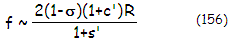while the appropriate shape factor q (obtained from the q factor for zero spherical aberration) is: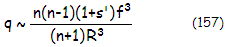Again, s' and c' are numerically negative in the Cassegrain. Needed lens radii are then obtained from Eq. 142-143, or from the following summary. A small secondary mirror location adjustment and slight correction of one of the two curves are usually sufficient to bring a system obtained through this procedure to a near-optimum level. Note that this calculation is for lower-order spherical aberration; as the primary relative aperture exceeds ~ƒ/2.5, higher-order spherical becomes significant with the standard, aplanatic Houghton corrector, and  will result in a discrepancy between results obtained for the lower-order aberration alone and the actual system error.EXAMPLE: 200mm ƒ/2.8/10.5 symmetrical-type BK7 corrector Houghton-Cassegrain (SPECS) with the primary-to-secondary separation 0.72ƒ1, thus k=0.28, secondary magnification m=4 (determining secondary radius of curvature in units of the primary's as ρ=0.373, and back focal distance in units of the primary focal length η=0.4) and the corrector-to-primary separation in units of the primary's radius of curvature σ=0.38. From Eq. 154, secondary-to-primary mirror spherical aberration ratio is s'=-0.328 (opposite in sign) and, from Eq. 155, the coma ratio is c'=-0.63. Substituting these two values into Eq. 156 gives the approximation of needed lens element focal length (absolute value) as ƒL~0.683R, R being the primary mirror radius of curvature. Needed lens shape factor for BK7 glass (n=1.519) is, from Eq. 157, q=0.067. It determines needed lens radii as R2,4~ 2(n-1)ƒL/(1-q)~0.76R and R1,3 = (1-q)R2,4/(1+q) = 0.66R.Ray trace shows that coma is corrected, but the system suffers from 0.15 wave RMS (546nm unit) of spherical aberration when the two lenses are in contact (1mm center separation). It is mainly the consequence of the higher-order aberration term not accounted for. Increasing the separation to 1.6mm cancels the lower-order contribution, reducing the error to 0.12 wave RMS of higher-order spherical aberration. Further increase in lens separation to 4mm induces as much of lower-order spherical needed to balance the combined aberration to 0.025 wave RMS. Similar effect is achieved keeping the lenses in contact by either by relaxing all corrector's radii by 0.7% (larger by a 1.007 factor), or to relax radii 1/3 to 746mm (absolute value), with no appreciable change in other aberrations. However, the increase in lens separation worsens longitudinal chromatism, which drops roughly at the level of a 4" ƒ/8-9 doublet achromat. In comparison, relaxing 1/3 radii while keeping lens separation at 1mm maintains near minimum chromatism, at the level of a 100mm ƒ/18 achromat.

Chromatism of the Houghton corrector can be reduced, while keeping it symmetrical radii-wise, either by opting for two-glass corrector, or by significantly relaxing the radii, at a price of re-introducing some coma. If, for instance, we opt for ƒL=|Re|, with the effective radius to correct Re=R/(1-s')1/3=-1279mm, due to to the primary's aberration being diminished by the secondary contribution (spherical aberration is inversely proportional to R3), the needed shape factor  q=n(n-1)ƒ3/(n+1)Re3=0.313.  This determines corrector radii (absolute values) as R2,4~ 2(n-1)ƒL/(1-q)~1932, and R1,3 = (1-q)R2,4/(1+q) =1011. The chromatism is more than twice smaller than the minimum in the standard arrangement, and can be considered negligible (the system is also somewhat slower, at ƒ/11). The coma increases to the diffraction-limited level (0.80 Strehl) at 5mm off-axis (0.3 degrees in diameter, or about 50% larger than in a comparable SCT with spherical mirrors).

Better redesigning option is to achromatize corrector. Just by switching the rear element glass from BK7 to BK8, slightly relaxing 3rd radius (from -739 to -745mm) and increasing lens separation to 1.7mm, chromatism is reduced nearly four times vs. minimum in the standard arrangement, without further optimization.

Larger relative secondary size generally reduces both, chromatism (due to greater offset of primary's spherical aberration and, therefore, weaker lens required) and coma (due to greater offset of the primary's coma).

Two-mirror Houghton system can also be arranged with a pair of plano-convex and plano-concave lenses (plano-symmetrical Houghton corrector) with identical curvatures (as illustrated on Fig. 108, right) of opposite signs. In this case, needed corrector lens radius (absolute value) for corrected 3rd order spherical aberration is, from Eq. 142, closely approximated by: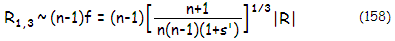Since for the corrector consisting of plano-lenses q equals 1 for both elements, and needed lens element focal length, absolute value, is approximated by ƒ~[(n+1)/n(n-1)(1+s')]1/3|R|, its coma coefficient is, from Eq. 138, approximated by: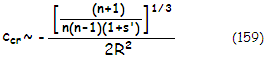Evidently, the s' parameter affects both, spherical aberration and coma of the corrector. With the primary mirror coma coefficient given by cp=(1-σ)/R2, the system coma aberration coefficient can be approximated by cs~ccr+(1+c')cp, and the resulting P-V wavefront error of coma at the best focus is:

W~csαD3/12          (160)

with α being the field angle in radians.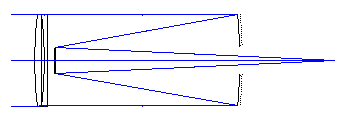EXAMPLE: 200mm ƒ/2.8/11 HCT with the identical optical configuration as above, only with plano-symmetrical BK7 Houghton corrector (n=1.519) instead of symmetrical, needs the lens element focal length, absolute value, |ƒ|~[(n+1)/n(n-1)(1+s*)]1/3|R|=1.68|R|, with the corresponding surface radii value |R1,3|=(n-1)|ƒ|=0.872|R|=977. With the two lenses in near-contact (5.5mm center separation), ray trace gives less than 1/50 wave P-V of spherical aberration, and nearly six times lower chromatism than the symmetrical corrector type aplanatic HCT telescope.

With s'=-0.33, the coma aberration coefficient for the corrector is ccr~-0.84/R2 which, with the mirror pair coma coefficient cm=(1+c')(1-σ)/R2=0.23/R2, gives the system coma coefficient as cs~ccr+(1+c')cp~-0.61/R2. At 0.1 degree off-axis, it results in the P-V wavefront error of coma W=0.00028mm, or 0.51 wave P-V for the e-line (546nm) - nearly 15% less than in the ƒ/2/10 commercial SCT. That nearly coincides with ray-trace results.

In general, the plano-symmetrical two-mirror Houghton system has coma comparable to that of an all-spherical (mirrors) SCT, which also can be cancelled or reduced by aspherizing the secondary (oblate ellipsoid), or by reducing stop separation.

Houghton-Cassegrain coma, as already mentioned, can be cancelled for any corrector location with the symmetrical corrector type, as well as asymmetrical aplanatic varieties. It is only a matter of generating exactly as much of the coma of opposite sign by the corrector to cancel that of the two mirrors (which is identical to that in the Schmidt-Cassegrain, given with Eq. 115). Calculating needed properties of the asymmetrical aplanatic Houghton corrector for two-mirror systems is based on the same principles as for the symmetrical types. It is matched against the combined spherical aberration and coma of the two mirrors, with the lens focal lengths and radii for zero coefficients obtained using Eq. 134 and Eq. 137.

Since Houghton corrector induces only negligible astigmatism, SCT relations for astigmatism and field curvature, Eq. 116-117, are applicable to the Houghton-Cassegrain as well.

Misalignment sensitivity of the Houghton-Cassegrain secondary is given by the general two-mirror system relations (Eq. 91.1-91.2). For the corrector itself, raytrace suggests sensitivity to decenter similar to that of the Schmidt corrector, and sensitivity to tilt about tenfold greater (similar to the tilt sensitivity of a full-aperture meniscus corrector). Sensitivity to despace is practically non-existent for all three corrector types. Sensitivity to despace between the two lens elements of the Houghton corrector is very forgiving, and so is the lens thickness tolerance.